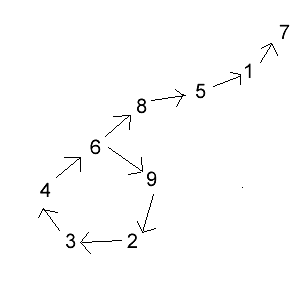#### You may also like### Consecutive Numbers

An investigation involving adding and subtracting sets of consecutive numbers. Lots to find out, lots to explore.### Calendar Capers

Choose any three by three square of dates on a calendar page...### Days and Dates

Investigate how you can work out what day of the week your birthday will be on next year, and the year after...

# 17s and 23s

##### Age 11 to 14 ShortChallenge Level

Multiples of $17$         Multiples of $23$
$17$                          $23$
$34$                          $46$
$51$                          $69$
$68$                          $92$
$85$

Can't use $17$ (except at the end) because none of the numbers start with $7$

Try starting from $34$:
$68\rightarrow85\rightarrow51\rightarrow17$   stuck (unless this is at the end)
$\nearrow$
$34\rightarrow46$
$\searrow$
$69\rightarrow92\rightarrow23\rightarrow34$   loop of all other numbers

The number will look like $...34692346923...$ and will go in loops or go into the 'stuck' sequence at the end

It could start with the $3,4,6,9$ or $2$ which gives $5$ options which are just loops.

It could go into the 'stuck' sequence at the end by going $8$ instead of $9$ after the last $6$. This works as long as $6$ isn't the last digit - so $4$ more options.

Total $9$ options.

This diagram shows how the numbers can be used.This problem is taken from the UKMT Mathematical Challenges.
You can find more short problems, arranged by curriculum topic, in our short problems collection.